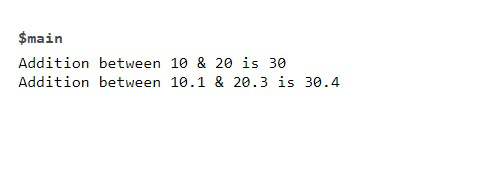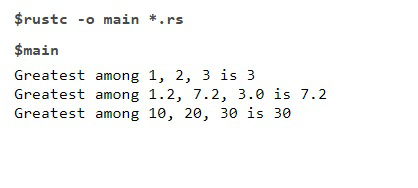GeeksforGeeks App
Open AppBrowser
Continue

# Rust – Generic Function

In Rust, Generic functions are very useful. Generic make code more flexible and provide more functionality to the callers of the function. It prevents code duplication as it is not required to define different functions of different types. Generics are specified in the signature of function where we actually specify the datatype of parameters and return type.

Let’s look into an example of normal functions:

fn sampleFunction1(varX:i32)->i32{

// this function accepts integer and return an integer

……………….

………………..

}

fn sampleFunction2(varX:f64)->f64{

……

……

// accepts float and return float

// same functionality of above function

}

Above are the two functions which are having same functionality but due to difference in the type of parameters and return type, two functions are defined. But the generic function will help us define the function once and use it with multiple types.

### Syntax:

```fn functionName<T>(variable_name1 : T, variable_name2 : T ,...) -> T {
......
......
}
where T represents the generic type. variable_name1,
variable_name2 are parameters of type generic.
The function will return a value of the same type T.
Let's look at the coding part of using a generic function.```

Let’s see another example to write a program to add two numbers of the same type in Rust.

Example 1:

## Rust

 `// Rust code for addition``fn` `main() {``    ``println!(``"Addition between 10 & 20 is {}"``, sumGeneric(10,20));``//30``    ``println!(``"Addition between 10.1 & 20.3 is {}"``, sumGeneric(10.1,20.3));``//30.4``}` `// generic function that add two numbers``use` `std::ops::Add;``fn` `sumGeneric + Copy> (a: T, b: T) -> T {``    ``return` `a + b;``}`

Output:If we didn’t used the generic function then we need to write two different functions where one function accepts integers and return an integer and other function that accepts floating point numbers and return floating point result. So here Generic function helps us to avoid code redundancy.

Let’s look at the another example of generic function for better understanding.

Example 2:

## Rust

 `// Rust program to find the largest number among 3 numbers.``fn` `main() {``    ``println!(``"Greatest among 1, 2, 3 is {}"``,greater(1,2,3));``    ``println!(``"Greatest among 1.2, 7.2, 3.0 is {}"``,greater(1.2,7.2,3.0));``    ``println!(``"Greatest among 10, 20, 30 is {}"``,greater(10,20,30));``}``fn` `greater(a:T,b:T,c:T)->T{``  ``if` `a>b && a>c{``    ``return` `a;``  ``}``  ``else` `if` `b>a && b>c{``    ``return` `b;``  ``}``  ``else``{``    ``return` `c;``  ``}``}`

Output:In the above code, inside the generic function we used PartialOrd represents the ordered trait that allows the parameters in the function to be compared. The advantage of using Generic function is it helps to prevent code duplication and make code looks more organized and concise.

My Personal Notes arrow_drop_up